# Writing and Graphing Linear Equations Linear equations can

• Slides: 58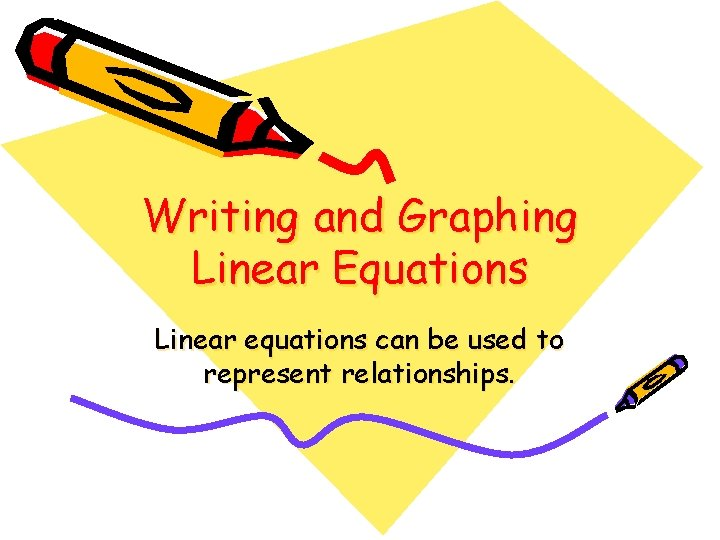Writing and Graphing Linear Equations Linear equations can be used to represent relationships.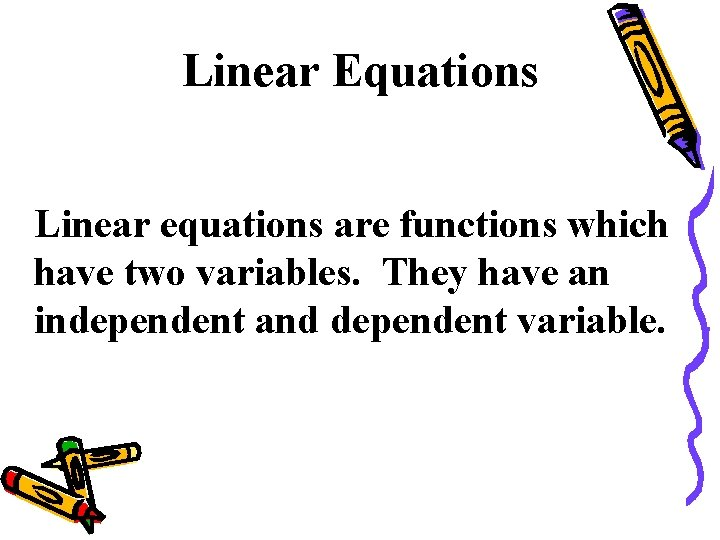Linear Equations Linear equations are functions which have two variables. They have an independent and dependent variable.Independent and Dependent Variables Independent Variables Dependent Variables Independent variables are variables that you put into the equations (usually x)! Dependent variables are variables that you solve for (usually y)!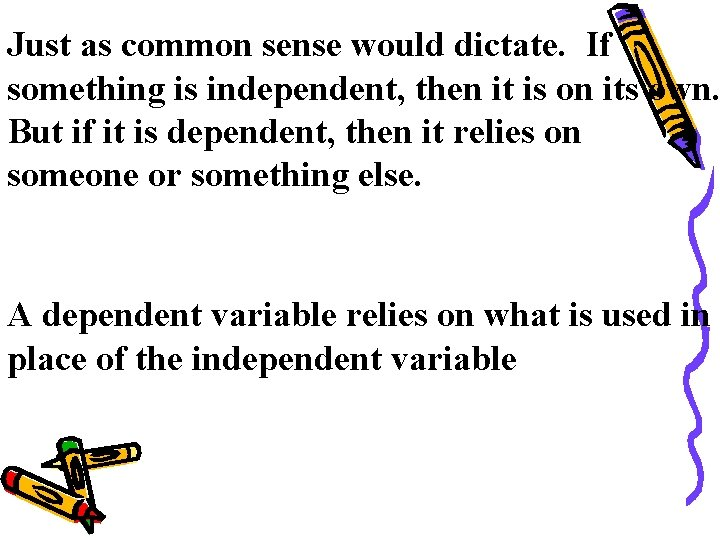Just as common sense would dictate. If something is independent, then it is on its own. But if it is dependent, then it relies on someone or something else. A dependent variable relies on what is used in place of the independent variabley = 2 x - 4 Using the equation above, y has to be the Dependent variable Why? Because it is the variable that is being solved for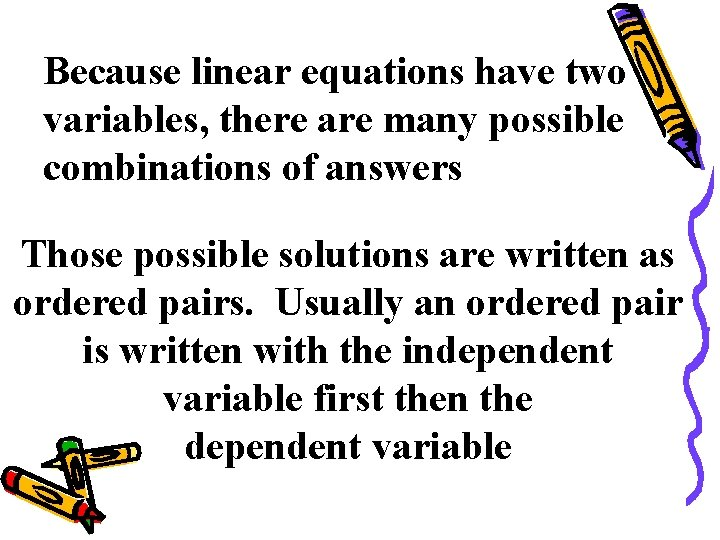Because linear equations have two variables, there are many possible combinations of answers Those possible solutions are written as ordered pairs. Usually an ordered pair is written with the independent variable first then the dependent variable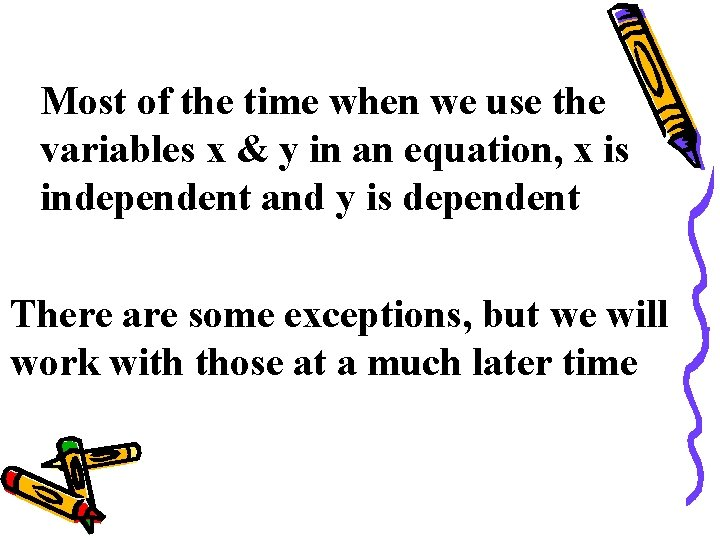Most of the time when we use the variables x & y in an equation, x is independent and y is dependent There are some exceptions, but we will work with those at a much later time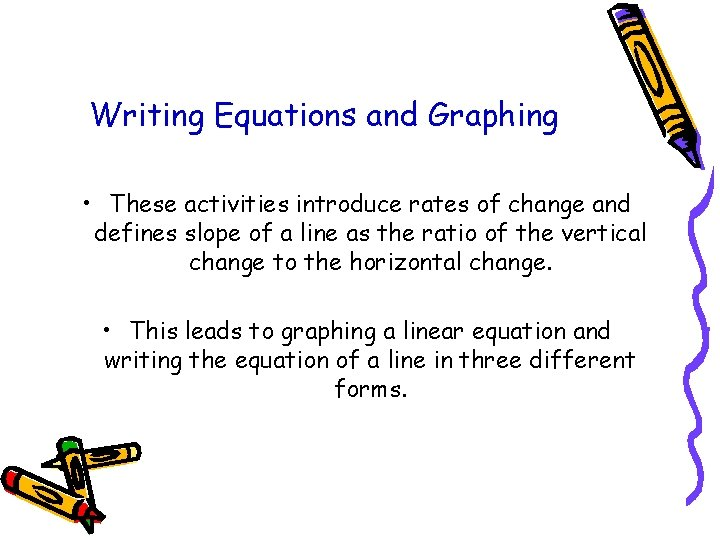Writing Equations and Graphing • These activities introduce rates of change and defines slope of a line as the ratio of the vertical change to the horizontal change. • This leads to graphing a linear equation and writing the equation of a line in three different forms.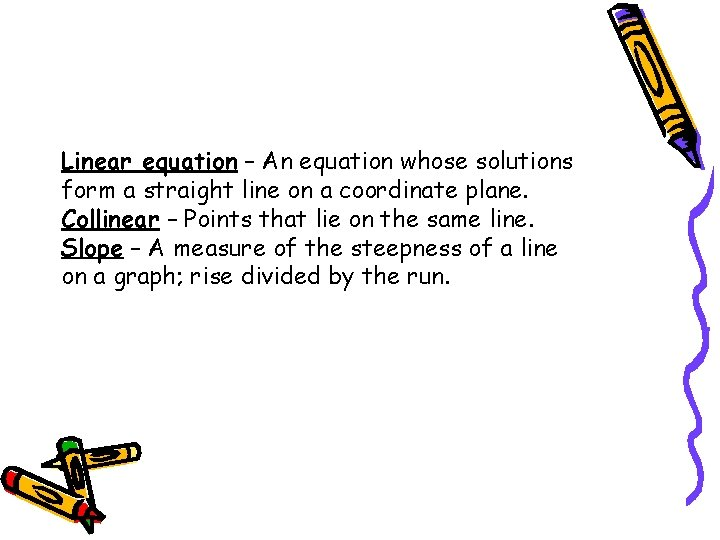Linear equation – An equation whose solutions form a straight line on a coordinate plane. Collinear – Points that lie on the same line. Slope – A measure of the steepness of a line on a graph; rise divided by the run.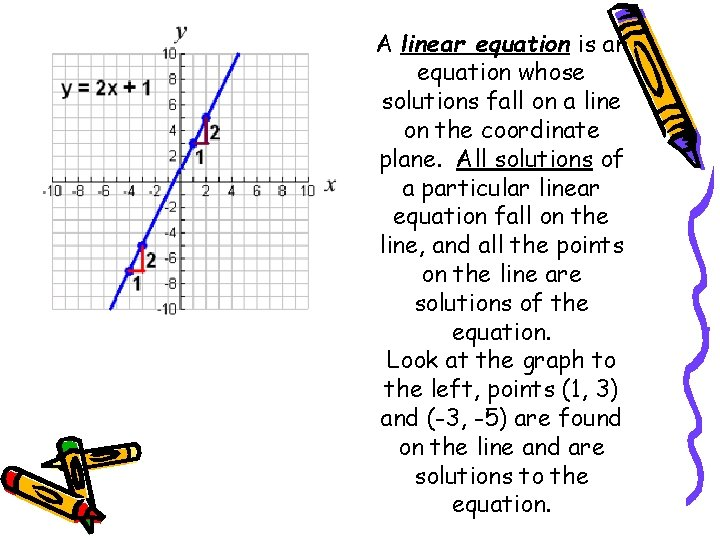A linear equation is an equation whose solutions fall on a line on the coordinate plane. All solutions of a particular linear equation fall on the line, and all the points on the line are solutions of the equation. Look at the graph to the left, points (1, 3) and (-3, -5) are found on the line and are solutions to the equation.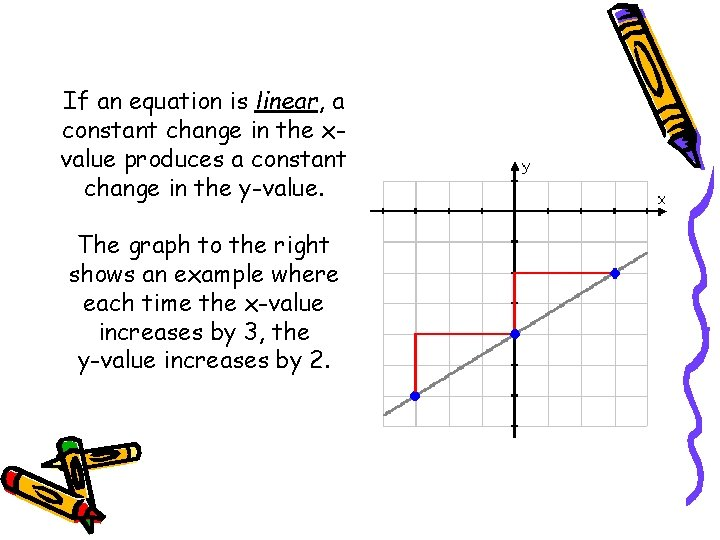If an equation is linear, a constant change in the xvalue produces a constant change in the y-value. The graph to the right shows an example where each time the x-value increases by 3, the y-value increases by 2.The equation y = 2 x + 6 is a linear equation because it is the graph of a straight line and each time x increases by 1 unit, y increases by 2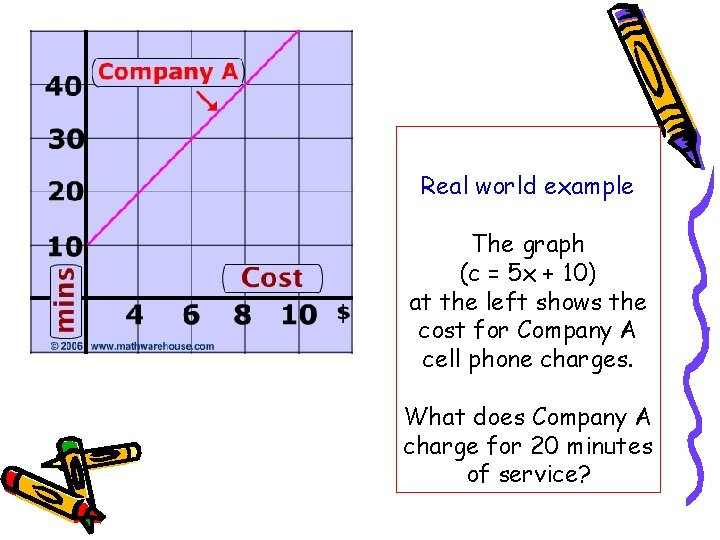Real world example The graph (c = 5 x + 10) at the left shows the cost for Company A cell phone charges. What does Company A charge for 20 minutes of service?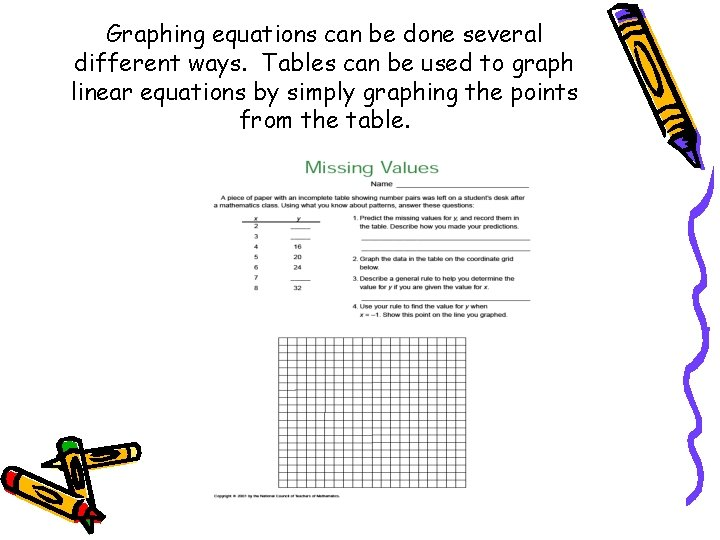Graphing equations can be done several different ways. Tables can be used to graph linear equations by simply graphing the points from the table.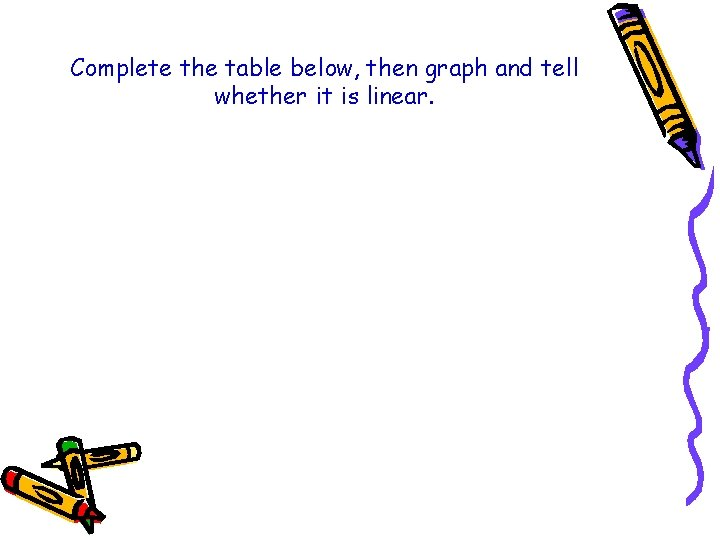Complete the table below, then graph and tell whether it is linear.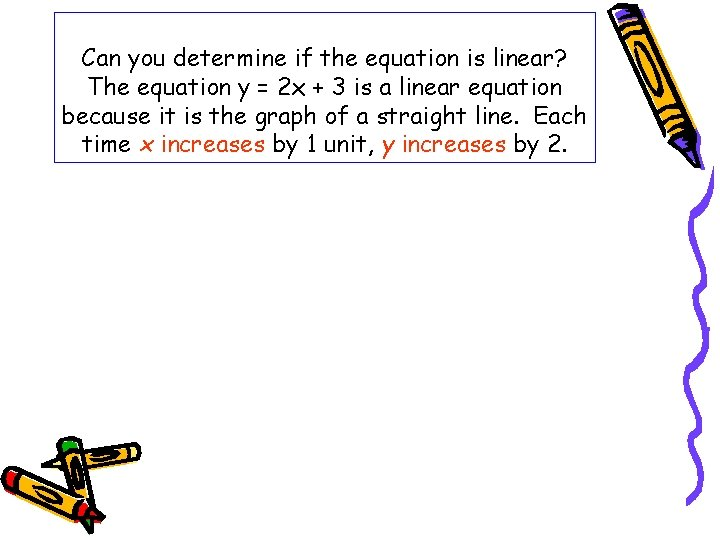Can you determine if the equation is linear? The equation y = 2 x + 3 is a linear equation because it is the graph of a straight line. Each time x increases by 1 unit, y increases by 2.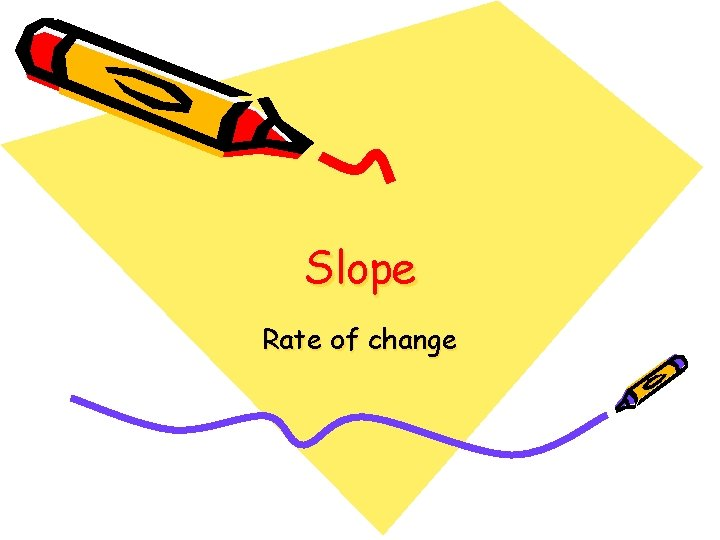Slope Rate of change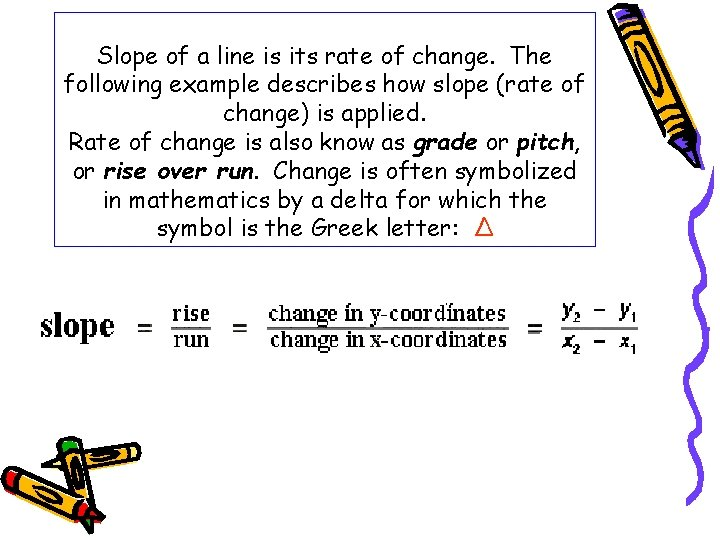Slope of a line is its rate of change. The following example describes how slope (rate of change) is applied. Rate of change is also know as grade or pitch, or rise over run. Change is often symbolized in mathematics by a delta for which the symbol is the Greek letter: ΔFinding slope (rate of change) using a graph and two points.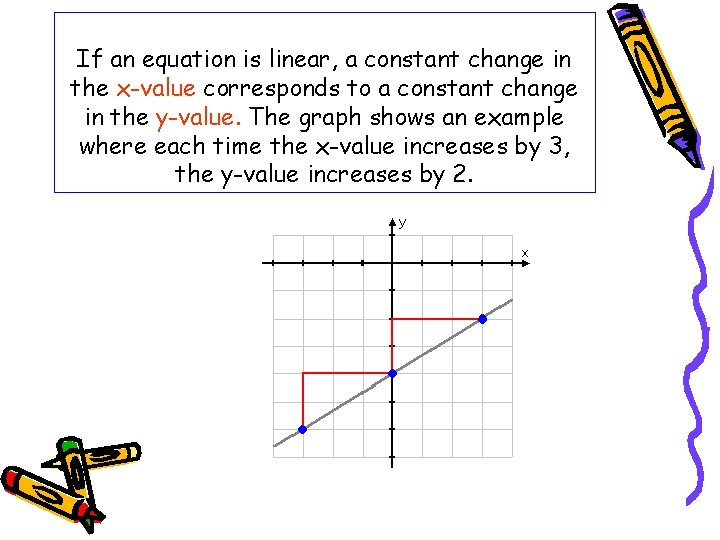If an equation is linear, a constant change in the x-value corresponds to a constant change in the y-value. The graph shows an example where each time the x-value increases by 3, the y-value increases by 2.Slopes: positive, negative, no slope (zero), undefined.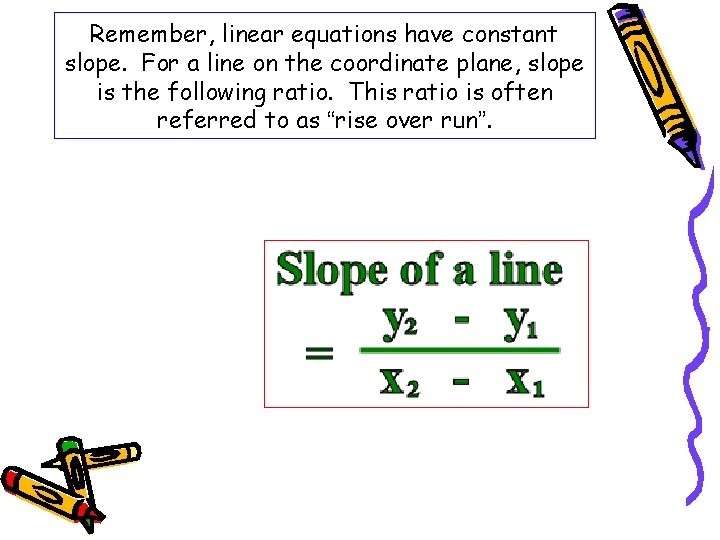Remember, linear equations have constant slope. For a line on the coordinate plane, slope is the following ratio. This ratio is often referred to as “rise over run”.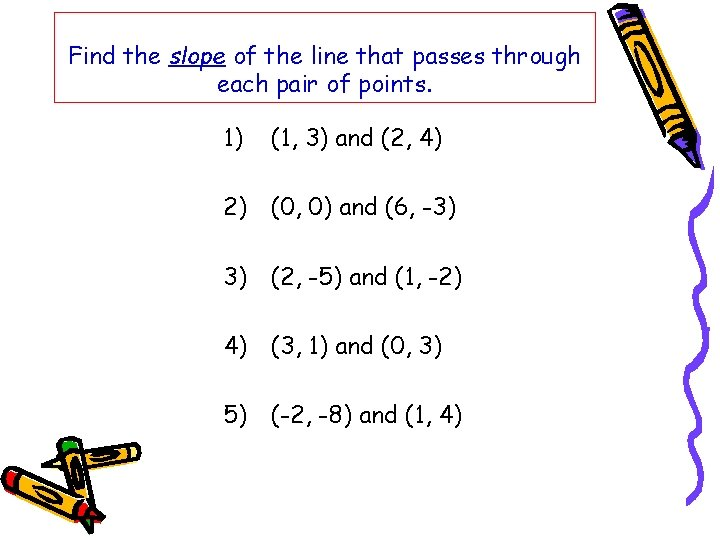Find the slope of the line that passes through each pair of points. 1) (1, 3) and (2, 4) 2) (0, 0) and (6, -3) 3) (2, -5) and (1, -2) 4) (3, 1) and (0, 3) 5) (-2, -8) and (1, 4)Graphing a Line Using a Point and the Slope Graph the line passing through (1, 3) with slope 2.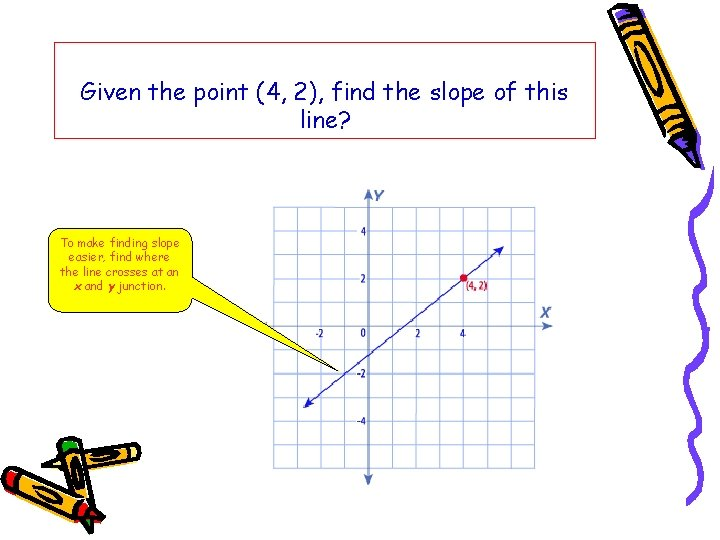Given the point (4, 2), find the slope of this line? To make finding slope easier, find where the line crosses at an x and y junction.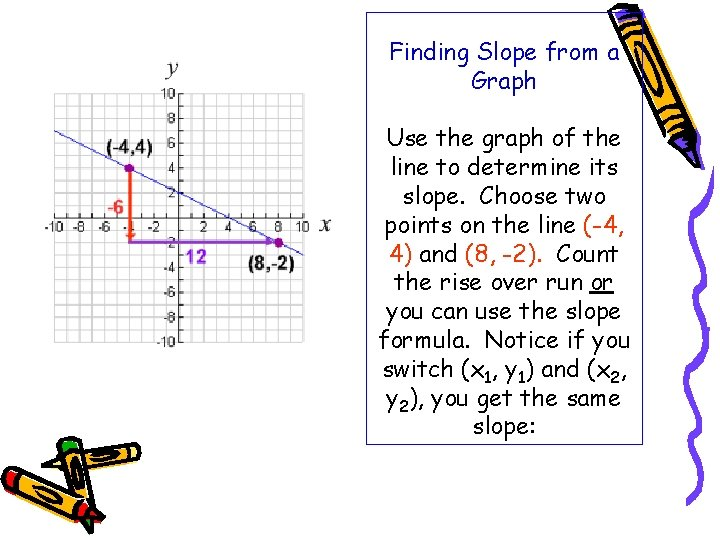Finding Slope from a Graph Use the graph of the line to determine its slope. Choose two points on the line (-4, 4) and (8, -2). Count the rise over run or you can use the slope formula. Notice if you switch (x 1, y 1) and (x 2, y 2), you get the same slope: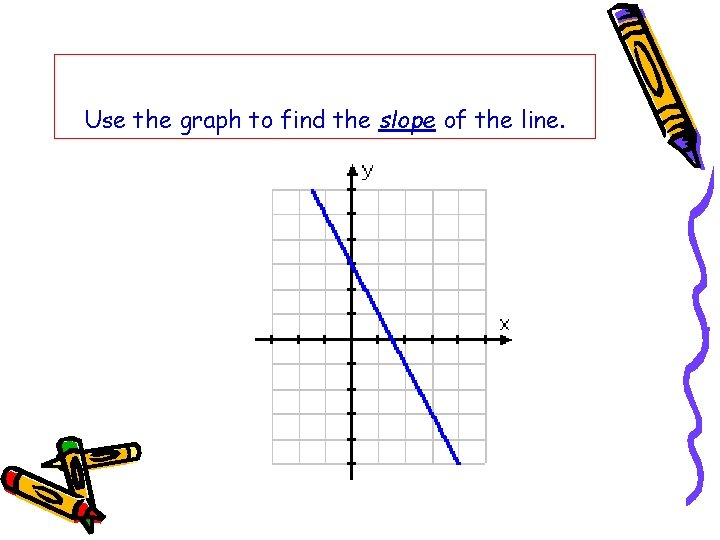Use the graph to find the slope of the line.Using Slopes and Intercepts x-intercepts and y-intercepts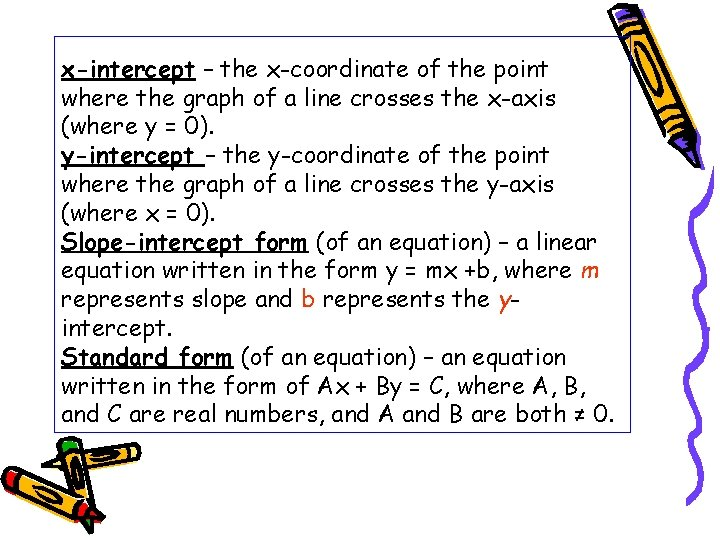x-intercept – the x-coordinate of the point where the graph of a line crosses the x-axis (where y = 0). y-intercept – the y-coordinate of the point where the graph of a line crosses the y-axis (where x = 0). Slope-intercept form (of an equation) – a linear equation written in the form y = mx +b, where m represents slope and b represents the yintercept. Standard form (of an equation) – an equation written in the form of Ax + By = C, where A, B, and C are real numbers, and A and B are both ≠ 0.• The standard form of a linear equation, you can use the x- and yintercepts to make a graph. • The x-intercept is the x-value of the point where the line crosses. • The y-intercept is the y-value of the point where the line crosses. Standard Form of an Equation Ax + By = C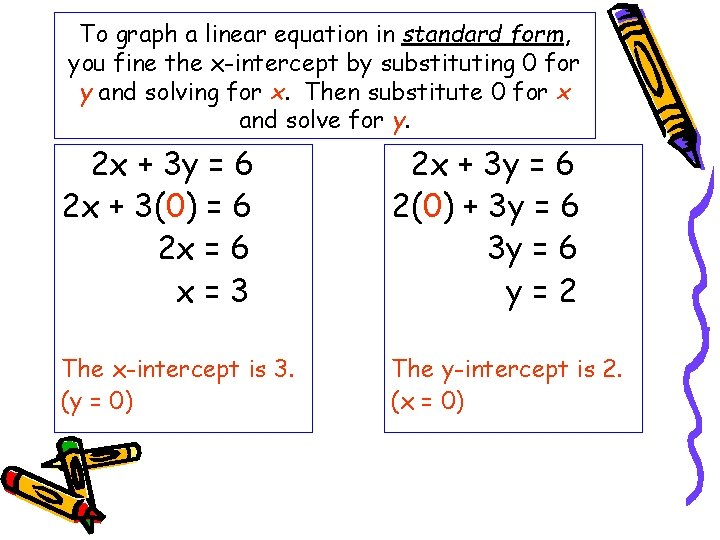To graph a linear equation in standard form, you fine the x-intercept by substituting 0 for y and solving for x. Then substitute 0 for x and solve for y. 2 x + 3 y = 6 2 x + 3(0) = 6 2 x = 6 x=3 2 x + 3 y = 6 2(0) + 3 y = 6 y=2 The x-intercept is 3. (y = 0) The y-intercept is 2. (x = 0)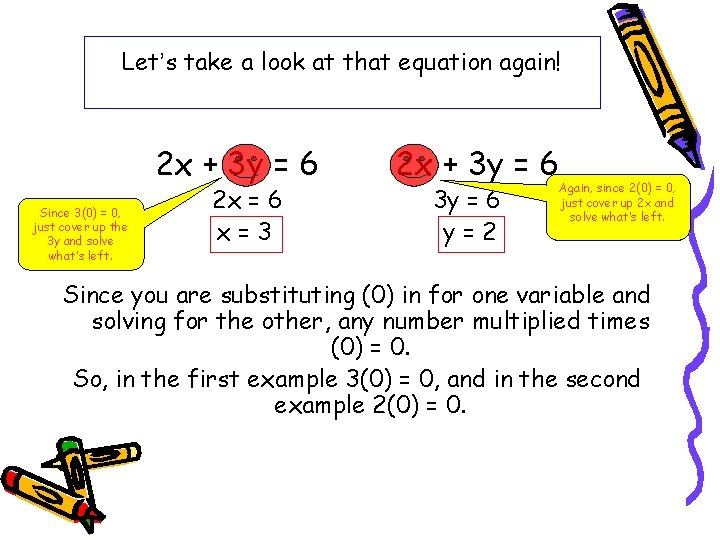Let’s take a look at that equation again! 2 x + 3 y = 6 Since 3(0) = 0, just cover up the 3 y and solve what’s left. 2 x = 6 x=3 2 x + 3 y = 6 y=2 Again, since 2(0) = 0, just cover up 2 x and solve what’s left. Since you are substituting (0) in for one variable and solving for the other, any number multiplied times (0) = 0. So, in the first example 3(0) = 0, and in the second example 2(0) = 0.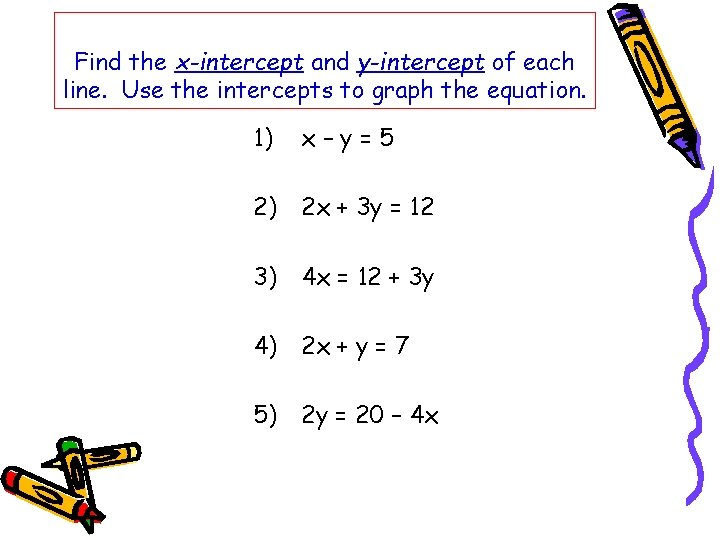Find the x-intercept and y-intercept of each line. Use the intercepts to graph the equation. 1) x–y=5 2) 2 x + 3 y = 12 3) 4 x = 12 + 3 y 4) 2 x + y = 7 5) 2 y = 20 – 4 x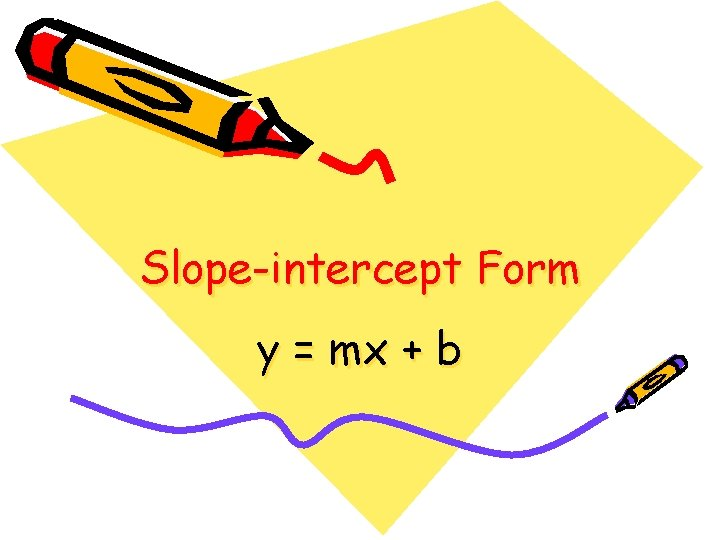Slope-intercept Form y = mx + b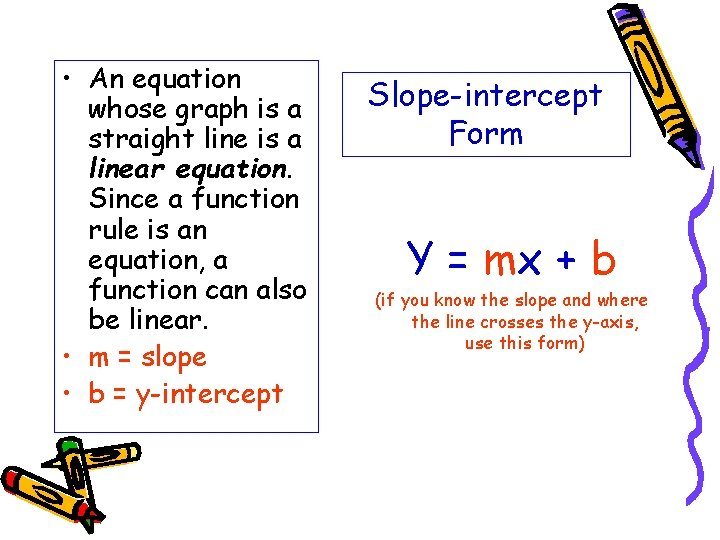• An equation whose graph is a straight line is a linear equation. Since a function rule is an equation, a function can also be linear. • m = slope • b = y-intercept Slope-intercept Form Y = mx + b (if you know the slope and where the line crosses the y-axis, use this form)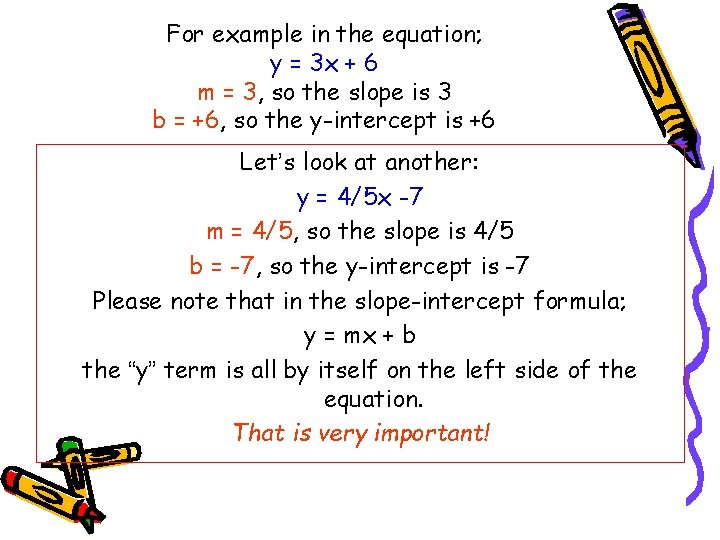For example in the equation; y = 3 x + 6 m = 3, so the slope is 3 b = +6, so the y-intercept is +6 Let’s look at another: y = 4/5 x -7 m = 4/5, so the slope is 4/5 b = -7, so the y-intercept is -7 Please note that in the slope-intercept formula; y = mx + b the “y” term is all by itself on the left side of the equation. That is very important!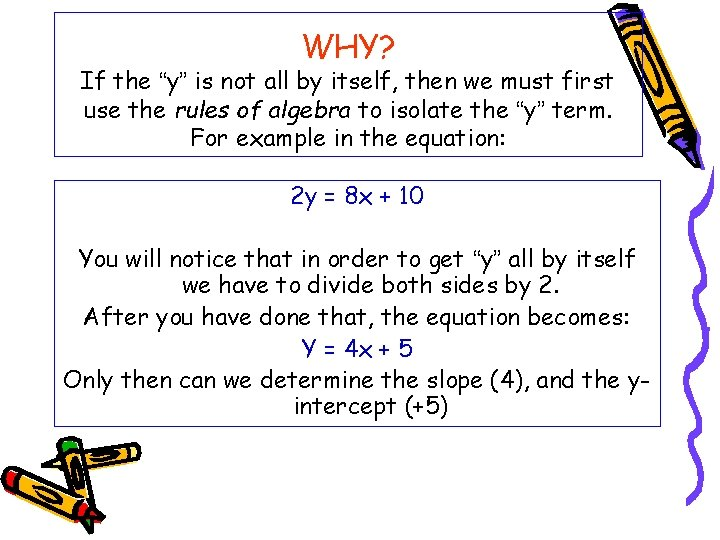WHY? If the “y” is not all by itself, then we must first use the rules of algebra to isolate the “y” term. For example in the equation: 2 y = 8 x + 10 You will notice that in order to get “y” all by itself we have to divide both sides by 2. After you have done that, the equation becomes: Y = 4 x + 5 Only then can we determine the slope (4), and the yintercept (+5)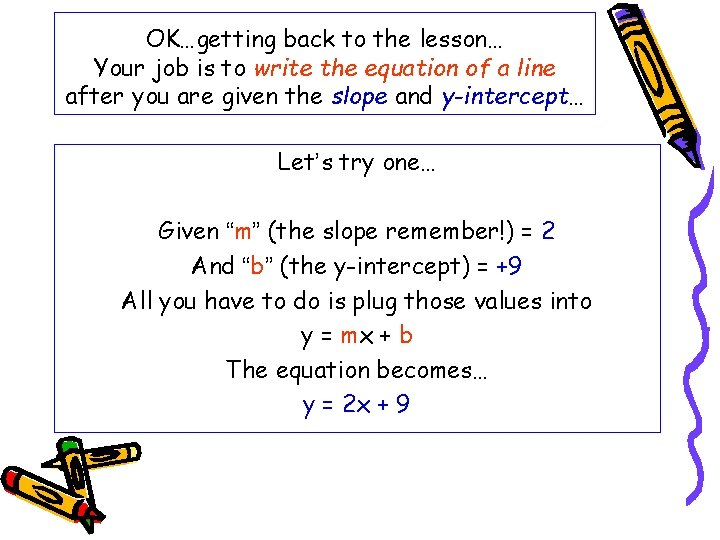OK…getting back to the lesson… Your job is to write the equation of a line after you are given the slope and y-intercept… Let’s try one… Given “m” (the slope remember!) = 2 And “b” (the y-intercept) = +9 All you have to do is plug those values into y = mx + b The equation becomes… y = 2 x + 9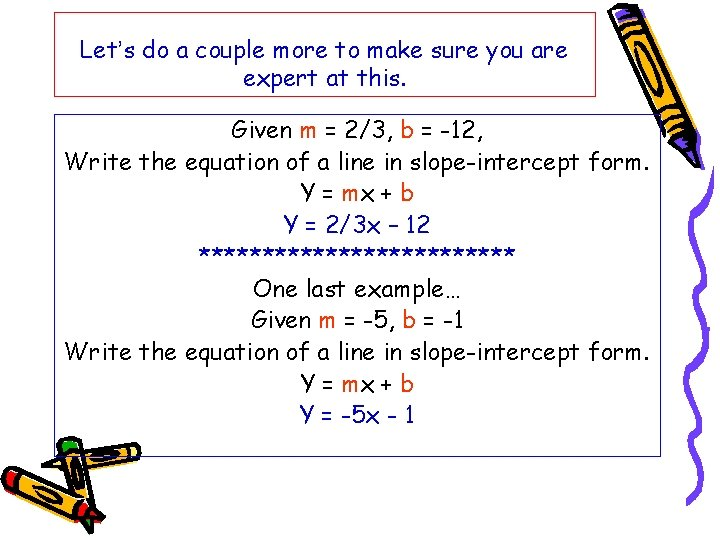Let’s do a couple more to make sure you are expert at this. Given m = 2/3, b = -12, Write the equation of a line in slope-intercept form. Y = mx + b Y = 2/3 x – 12 ************* One last example… Given m = -5, b = -1 Write the equation of a line in slope-intercept form. Y = mx + b Y = -5 x - 1Given the slope and y-intercept, write the equation of a line in slope-intercept form. 1) m = 3, b = -14 2) m = -½, b = 4 3) m = -3, b = -7 4) m = 1/2 , b = 0 5) m = 2, b = 4 Slope-intercept form of an equation Y = mx + bUsing slope-intercept form to find slopes and y-intercepts The graph at the right shows the equation of a line both in standard form and slope-intercept form. You must rewrite the equation 6 x – 3 y = 12 in slope-intercept to be able to identify the slope and yintercept.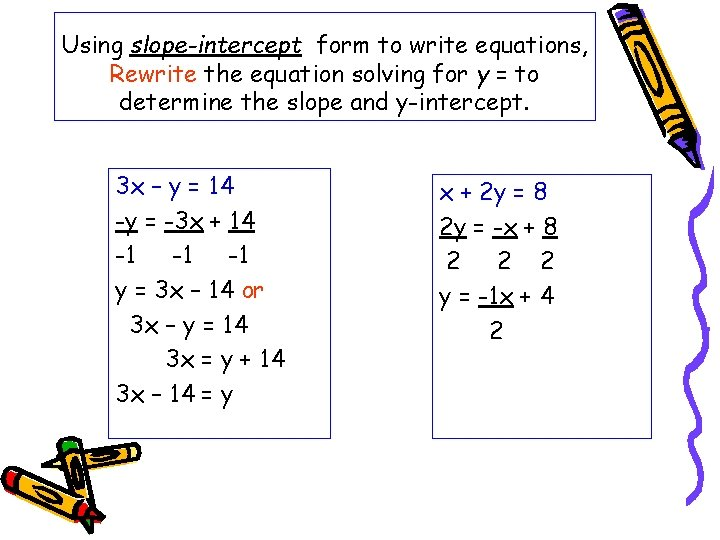Using slope-intercept form to write equations, Rewrite the equation solving for y = to determine the slope and y-intercept. 3 x – y = 14 -y = -3 x + 14 -1 -1 -1 y = 3 x – 14 or 3 x – y = 14 3 x = y + 14 3 x – 14 = y x + 2 y = 8 2 y = -x + 8 2 2 2 y = -1 x + 4 2Write each equation in slope-intercept form. Identify the slope and y-intercept. 2 x + y = 10 -4 x + y = 6 4 x + 3 y = 9 2 x + y = 3 5 y = 3 x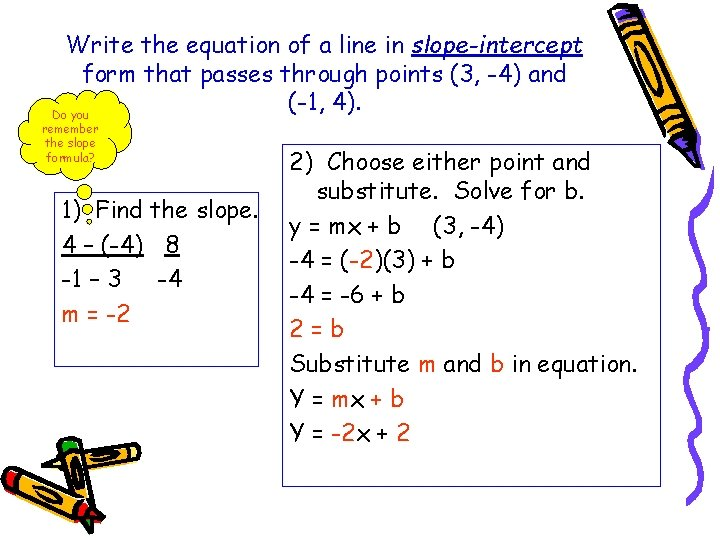Write the equation of a line in slope-intercept form that passes through points (3, -4) and (-1, 4). Do you remember the slope formula? 1) Find the slope. 4 – (-4) 8 -1 – 3 -4 m = -2 2) Choose either point and substitute. Solve for b. y = mx + b (3, -4) -4 = (-2)(3) + b -4 = -6 + b 2=b Substitute m and b in equation. Y = mx + b Y = -2 x + 2Write the equation of the line in slope-intercept form that passes through each pair of points. 1) (-1, -6) and (2, 6) 2) (0, 5) and (3, 1) 3) (3, 5) and (6, 6) 4) (0, -7) and (4, 25) 5) (-1, 1) and (3, -3)Point-Slope Form Writing an equation when you know a point and the slope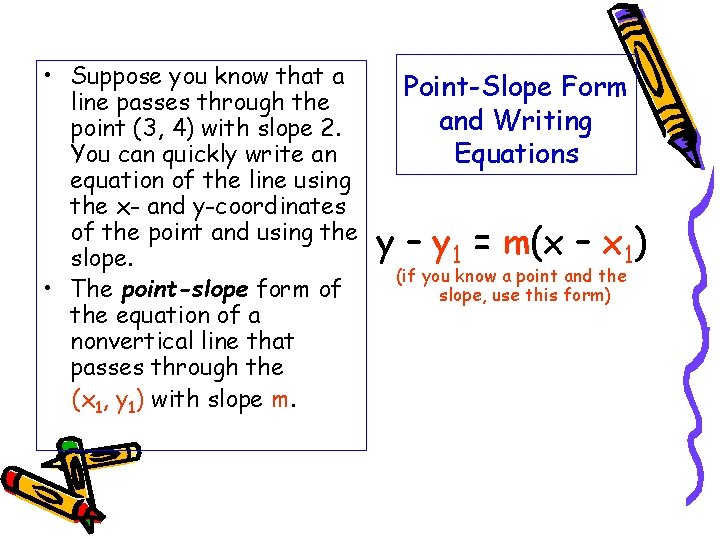• Suppose you know that a line passes through the point (3, 4) with slope 2. You can quickly write an equation of the line using the x- and y-coordinates of the point and using the slope. • The point-slope form of the equation of a nonvertical line that passes through the (x 1, y 1) with slope m. Point-Slope Form and Writing Equations y – y 1 = m(x – x 1) (if you know a point and the slope, use this form)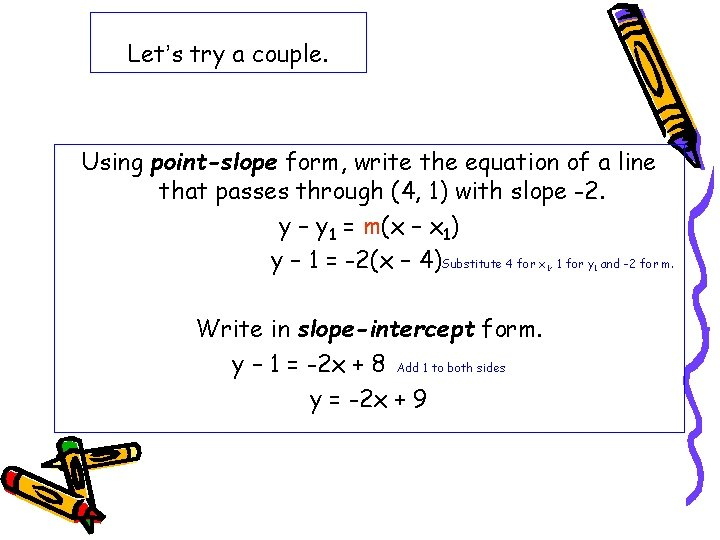Let’s try a couple. Using point-slope form, write the equation of a line that passes through (4, 1) with slope -2. y – y 1 = m(x – x 1) y – 1 = -2(x – 4) Substitute 4 for x , 1 for y and -2 for m. 1 Write in slope-intercept form. y – 1 = -2 x + 8 Add 1 to both sides y = -2 x + 9 1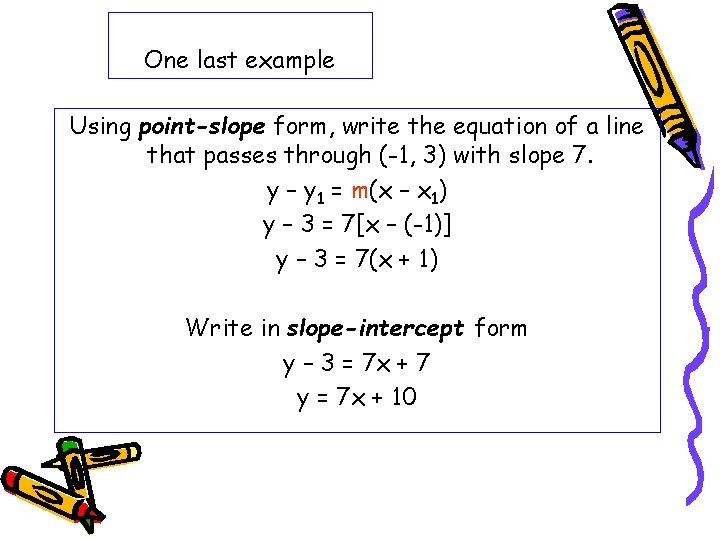One last example Using point-slope form, write the equation of a line that passes through (-1, 3) with slope 7. y – y 1 = m(x – x 1) y – 3 = 7[x – (-1)] y – 3 = 7(x + 1) Write in slope-intercept form y – 3 = 7 x + 7 y = 7 x + 10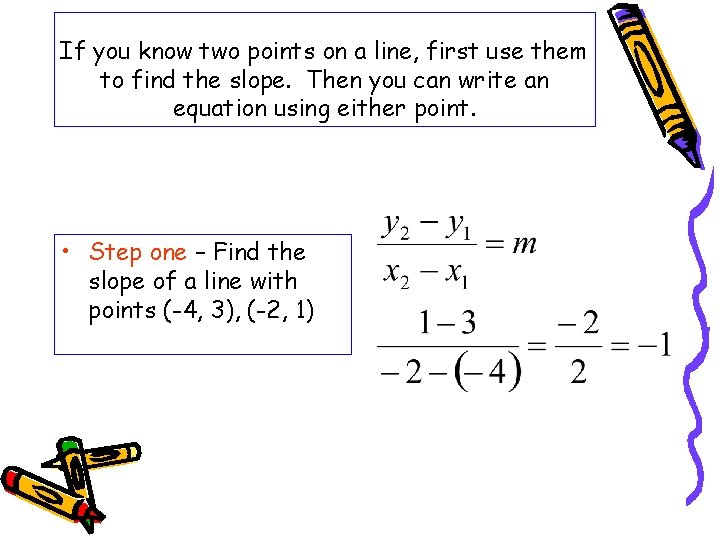If you know two points on a line, first use them to find the slope. Then you can write an equation using either point. • Step one – Find the slope of a line with points (-4, 3), (-2, 1)Step Two – Use either point to write the equation in point-slope form. Use (-4, 3) y – y 1 = m(x – x 1) Y – 3 = -1[x – (-4)] Y – 3 = -1(x + 4) Write in slope-intercept form Y – 3 = -1(x + 4) Y – 3 = -x - 4 Y = -x - 1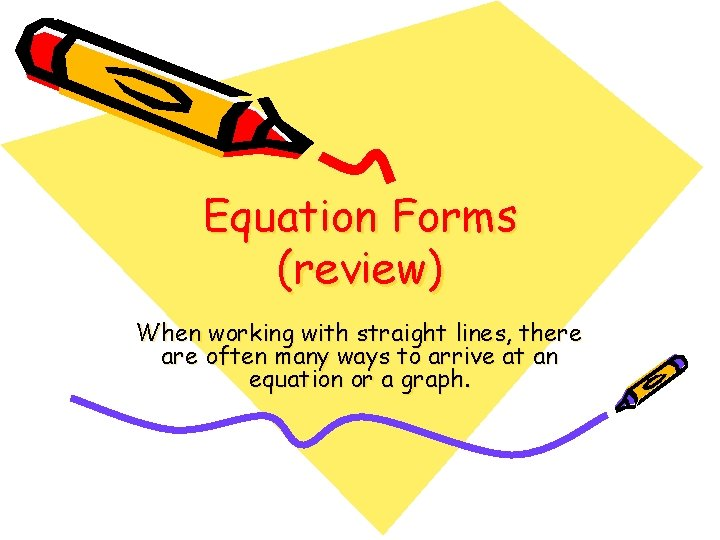Equation Forms (review) When working with straight lines, there are often many ways to arrive at an equation or a graph.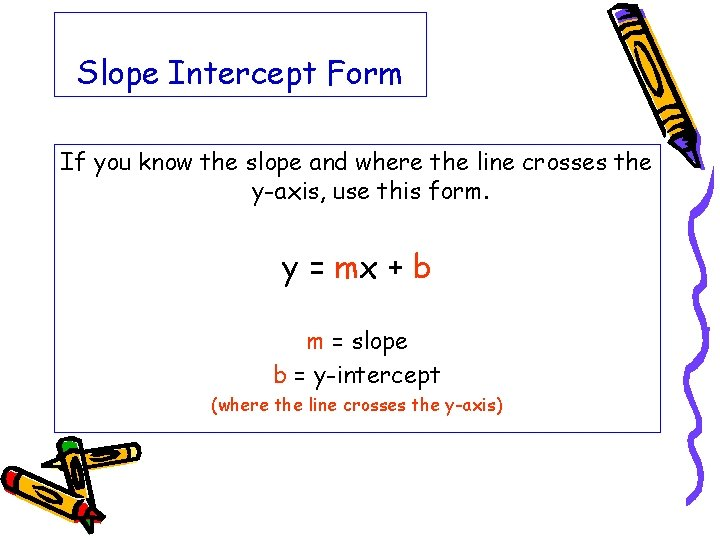Slope Intercept Form If you know the slope and where the line crosses the y-axis, use this form. y = mx + b m = slope b = y-intercept (where the line crosses the y-axis)Point Slope Form If you know a point and the slope, use this form. y – y 1 = m(x – x 1) m = slope (x 1, y 1) = a point on the lineHorizontal Lines y=3 (or any number) Lines that are horizontal have a slope of zero. They have “run” but no “rise”. The rise/run formula for slope always equals zero since rise = o. y = mx + b y = 0 x + 3 y=3 This equation also describes what is happening to the y-coordinates on the line. In this case, they are always 3.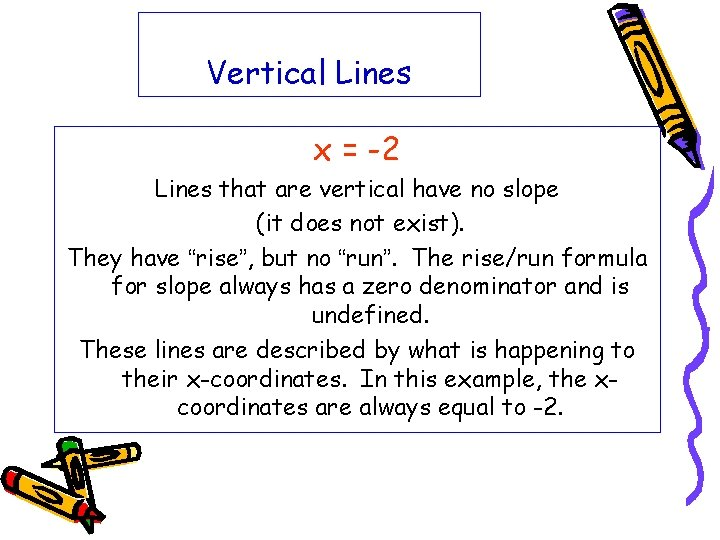Vertical Lines x = -2 Lines that are vertical have no slope (it does not exist). They have “rise”, but no “run”. The rise/run formula for slope always has a zero denominator and is undefined. These lines are described by what is happening to their x-coordinates. In this example, the xcoordinates are always equal to -2.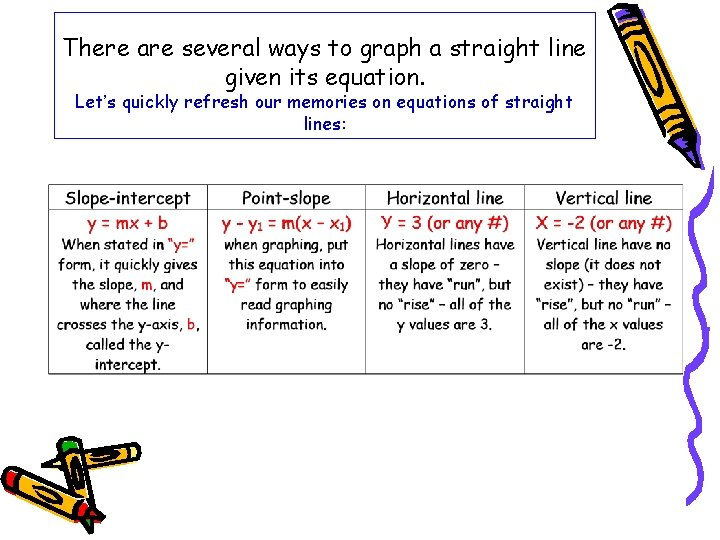There are several ways to graph a straight line given its equation. Let’s quickly refresh our memories on equations of straight lines: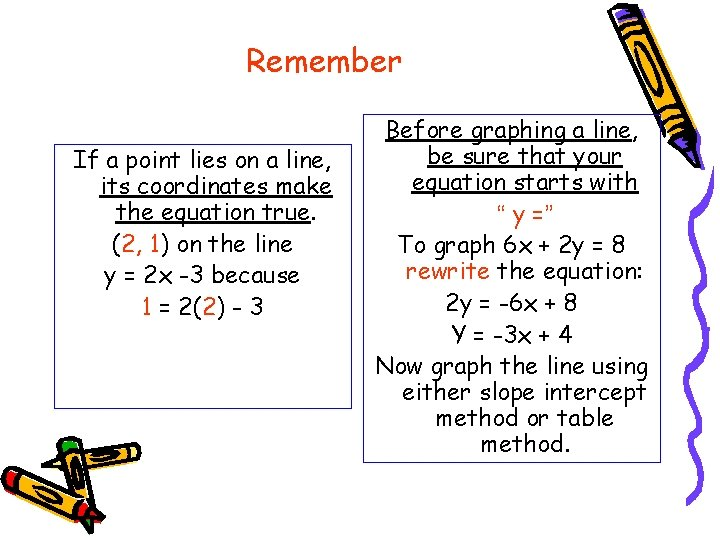Remember If a point lies on a line, its coordinates make the equation true. (2, 1) on the line y = 2 x -3 because 1 = 2(2) - 3 Before graphing a line, be sure that your equation starts with “ y =” To graph 6 x + 2 y = 8 rewrite the equation: 2 y = -6 x + 8 Y = -3 x + 4 Now graph the line using either slope intercept method or table method.